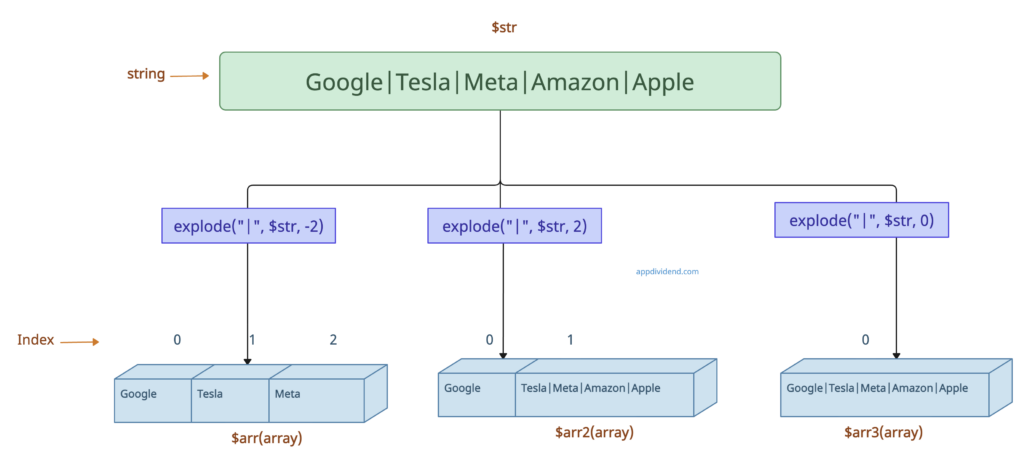# PHP explode() Function

PHP explode() function is “used to split a string into an array.” It splits a string based on a specified delimiter.

## Syntax

``````explode(separator,\$string,limit)
``````

## Parameters

separator(required): The boundary string.

\$string(required): The input string to split.

limit(optional): It specifies the number of array elements to return. This can be any integer.

• Positive: If a positive value is passed as the limit parameter, the returned array will contain a maximum of ‘limit’ elements, with the last element containing the rest of the string.
• Negative: If a negative value is passed as the limit parameter, the returned array will contain all components except for the last -limit are returned.
• Zer0: If a zero value is passed as the limit parameter, the returned array will consist of a single element containing the entire string.

## Return value

It returns an array of strings.

## Example 1: How to Use explode() function

``````<?php

\$str = "Google Tesla Meta Amazon Apple";
\$arr = explode(" ", \$str);
print_r(\$arr);
``````

Output

``````Array
(
 => Tesla
 => Meta
 => Amazon
 => Apple
)``````

## Example 2: Passing limit parameter``````<?php

\$arr = explode("|", \$str, -2);
\$arr2 = explode("|", \$str, 2);
\$arr3 = explode("|", \$str, 0);

echo "negative :"."\n";
print_r(\$arr)."\n";

echo "positive :"."\n";
print_r(\$arr2)."\n";

echo "zero :"."\n";
print_r(\$arr3)."\n";
``````

Output

``````negative :
Array
(
 => Tesla
 => Meta
)
positive :
Array
(
 => Tesla|Meta|Amazon|Apple
)
zero :
Array
(
)``````

## Example 3: Split empty string

``````<?php

\$str = "";
\$arr = explode(",", \$str);
print_r(\$arr);
``````

Output

``````Array
(
 =>
)``````

If you split the empty string, you get the one-element array with 0 as the key and the empty string for the value.

That’s it.

Categories PHP

This site uses Akismet to reduce spam. Learn how your comment data is processed.